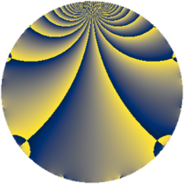# Properties

 Label 2850.2.fLevel $2850$ Weight $2$ Character orbit 2850.f Rep. character $\chi_{2850}(2051,\cdot)$ Character field $\Q$ Dimension $128$ Sturm bound $1200$

# Learn more about

## Defining parameters

 Level: $$N$$ $$=$$ $$2850 = 2 \cdot 3 \cdot 5^{2} \cdot 19$$ Weight: $$k$$ $$=$$ $$2$$ Character orbit: $$[\chi]$$ $$=$$ 2850.f (of order $$2$$ and degree $$1$$) Character conductor: $$\operatorname{cond}(\chi)$$ $$=$$ $$57$$ Character field: $$\Q$$ Sturm bound: $$1200$$

## Dimensions

The following table gives the dimensions of various subspaces of $$M_{2}(2850, [\chi])$$.

Total New Old
Modular forms 624 128 496
Cusp forms 576 128 448
Eisenstein series 48 0 48

## Trace form

 $$128q + 128q^{4} - 2q^{6} - 12q^{7} + 2q^{9} + O(q^{10})$$ $$128q + 128q^{4} - 2q^{6} - 12q^{7} + 2q^{9} + 128q^{16} - 24q^{19} - 2q^{24} - 12q^{28} + 2q^{36} + 30q^{39} + 10q^{42} + 172q^{49} - 8q^{54} - 14q^{57} + 12q^{58} - 64q^{61} + 62q^{63} + 128q^{64} - 8q^{66} + 28q^{73} - 24q^{76} + 66q^{81} + 42q^{87} + 4q^{93} - 2q^{96} + 40q^{99} + O(q^{100})$$

## Decomposition of $$S_{2}^{\mathrm{new}}(2850, [\chi])$$ into newform subspaces

The newforms in this space have not yet been added to the LMFDB.

## Decomposition of $$S_{2}^{\mathrm{old}}(2850, [\chi])$$ into lower level spaces

$$S_{2}^{\mathrm{old}}(2850, [\chi]) \cong$$ $$S_{2}^{\mathrm{new}}(57, [\chi])$$$$^{\oplus 6}$$$$\oplus$$$$S_{2}^{\mathrm{new}}(114, [\chi])$$$$^{\oplus 3}$$$$\oplus$$$$S_{2}^{\mathrm{new}}(285, [\chi])$$$$^{\oplus 4}$$$$\oplus$$$$S_{2}^{\mathrm{new}}(570, [\chi])$$$$^{\oplus 2}$$$$\oplus$$$$S_{2}^{\mathrm{new}}(1425, [\chi])$$$$^{\oplus 2}$$